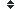volume (124 synonym found)

### Sort volume by:    Alphabetically| Length| Random | DefinitionDefinition of volume:

(n) : a unit of three dimensional measure of space that comprises a length, a width and a height. It is measured in units of cubic centimeters in metric, cubic inches or cubic feet in English measurement. (The room is 9x12x8, so its volume is 864 cubic feet.); strength of sound. Measured in decibels. (Please turn down the volume on the stereo.)
History
This word has been
viewed 3,613 times.

Related searches
for volume:

Most Recent

Most Popular

Definition of volume:

(n) : a unit of three dimensional measure of space that comprises a length, a width and a height. It is measured in units of cubic centimeters in metric, cubic inches or cubic feet in English measurement. (The room is 9x12x8, so its volume is 864 cubic feet.); strength of sound. Measured in decibels. (Please turn down the volume on the stereo.)